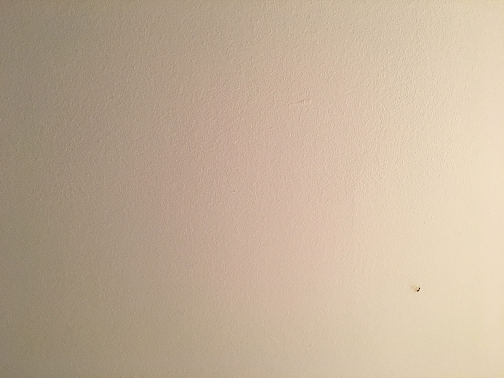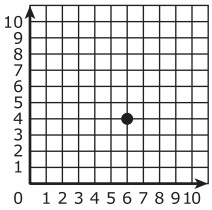# Patterns and the Coordinate Plane

## Objective

Construct a coordinate plane and identify the coordinates of given points.

## Common Core Standards

### Core Standards

?

• 5.G.A.1 — Use a pair of perpendicular number lines, called axes, to define a coordinate system, with the intersection of the lines (the origin) arranged to coincide with the 0 on each line and a given point in the plane located by using an ordered pair of numbers, called its coordinates. Understand that the first number indicates how far to travel from the origin in the direction of one axis, and the second number indicates how far to travel in the direction of the second axis, with the convention that the names of the two axes and the coordinates correspond (e.g., x-axis and x-coordinate, y-axis and y-coordinate).

?

• 2.MD.B.6

## Criteria for Success

?

1. Understand the need for a system in which a point’s location can be described in two dimensions.
2. Understand that the coordinate plane is a plane in which a point is represented using two coordinates that determine the precise location of that point (MP.6).
3. Understand that a coordinate plane is defined by two perpendicular lines, called the x-axis and the y-axis, that intersect at the origin, which coincides with the zero on each number line.
4. Understand that the location of a point in the coordinate plane is defined by its x-coordinate and its y-coordinate, which correspond with how far a point is in the direction of the x-axis and y-axis, respectively.
5. Given a point in a coordinate plane with intervals of 1 unit, determine its location, and write it as a coordinate pair, which is a type of ordered pair

## Tips for Teachers

?

• The following materials are needed for today's lesson: grid paper Markers or crayons
• You can print copies of this Inch Grid Paper if you do not have grid paper already.
• Even when lessons do not call for it, students may benefit from using grid paper to aid in their precision (MP.6), though they may not need it. Make it available for students to use but without explicitly requiring the use of the tool, allowing them to use appropriate tools strategically (MP.5).
• An ordered pair is a pair of two things written in a certain order. A coordinate pair is a pair of two coordinates written in a certain order, x then y. This distinction is not important for Grade 5 students and thus the terms are used interchangeably throughout the unit.

#### Remote Learning Guidance

If you need to adapt or shorten this lesson for remote learning, we suggest prioritizing Anchor Tasks 2 and 3 (benefit from worked examples). You could consolidate these to be a single Anchor Task. Find more guidance on adapting our math curriculum for remote learning here.

#### Fishtank Plus

• Problem Set
• Student Handout Editor
• Vocabulary Package

?

### Problem 1

Mr. Ingall, Mrs. Ingall’s husband, spotted a fly on the wall in their house. He wanted to catch it and let it free outside, but he hates bugs. How should he describe to Mrs. Ingall where the fly is on the wall so that she can catch it?### Problem 2

Construct a coordinate plane on grid paper.

### Problem 3

Determine the location of the shapes on the following coordinate plane and write them as an ordered pair:1. Checkmark: __________
2. Square: __________
3. Star: __________
4. Triangle: __________
5. Circle: __________

#### References

EngageNY Mathematics Grade 5 Mathematics > Module 6 > Topic A > Lesson 2Concept Development

Grade 5 Mathematics > Module 6 > Topic A > Lesson 2 of the New York State Common Core Mathematics Curriculum from EngageNY and Great Minds. © 2015 Great Minds. Licensed by EngageNY of the New York State Education Department under the CC BY-NC-SA 3.0 US license. Accessed Dec. 2, 2016, 5:15 p.m..

Modified by The Match Foundation, Inc.

## Problem Set & Homework

#### Discussion of Problem Set

• I think that point L has coordinates (1, 3)  in #1. What mistake did I make?
• Share your thinking about #4. What did we learn today that could help the pirate locate his treasure more easily?
• What new math vocabulary did we learn today?
• Why is a vertical line at the origin the best place for the y-axis?
• Why would it be important for us to all follow the same order when we write down the x-axis and y-coordinates?
• Grid paper is sometimes used when working on the coordinate plane. How is grid paper helpful in working on the coordinate plane?

?

### Problem 1

Select the three statements that correctly describe the point plotted on the coordinate plane.A. The point is located at the ordered pair (4, 6).

B. The point is located at the ordered pair (6, 4).

C. The x-coordinate is 6 and the y-coordinate is 4.

D. The x-coordinate is 4 and the y-coordinate is 6.

E. The point is 4 units to the right of the origin on the x-axis and 6 units up from the origin on the y-axis.

F. The point is 6 units to the right of the origin on the x-axis and 4 units up from the origin on the y-axis.

#### References

PARCC Mathematics Practice Tests Grade 5 Mathematics Paper-Based Practice TestQuestion #23

### Problem 2

On the coordinate plane above, highlight each of the following features with a marker or colored pencil:

1. Highlight the origin in red.
2. Highlight the x-axis in yellow.
3. Highlight the y-axis in blue.

### Mastery Response

?